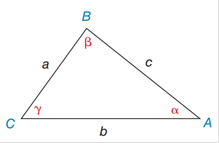Chapter 11.4, Problem 6E### Elementary Geometry for College St...

6th Edition
Daniel C. Alexander + 1 other
ISBN: 9781285195698

#### Solutions

Chapter
Section### Elementary Geometry for College St...

6th Edition
Daniel C. Alexander + 1 other
ISBN: 9781285195698
Textbook Problem
3 views

# In Exercises 5 and 6, state the form of the Law of Cosines used to solve the problem. Using the values provided, give the answer in a form such as a 2 = b 2 + c 2 − 2 a b   cos α . See the figure for Exercises 1 - 8.a) Find b if it is known that a = 5.7 ,   c = 8.2  and  β = 79 ∘ .b) Find β if it is known that a = 6 ,   b = 8 ,  and  c = 9 .Exercises 1-8

To determine

a.

To Find:

The side of b by Law of Cosines.

Explanation

Formula:

The Law of Cosines

a2=b2+c22bccosα

b2=a2+c22accosβ

c2=a2+b22abcosγ

Where α, β, and γ is the acute angle of the triangle, and a, b, and c is the sides of the triangle.

Calculation:

Given,

In ABC

a=5.7

c=8.2 and

β=79

By the Law of Cosines:

b2=a2+c22accosβ

Substitute a=5

To determine

b.

To Find:

The angle of β by using the Law of Cosines.

### Still sussing out bartleby?

Check out a sample textbook solution.

See a sample solution

#### The Solution to Your Study Problems

Bartleby provides explanations to thousands of textbook problems written by our experts, many with advanced degrees!

Get Started

#### Evaluate the integral, if it exists. sectan1+secd

Single Variable Calculus: Early Transcendentals, Volume I

#### In Exercises 13-20, sketch a set of coordinate axes and plot each point. 18. (52,32)

Applied Calculus for the Managerial, Life, and Social Sciences: A Brief Approach

#### For a given , the corresponding δ in the definition of is:

Study Guide for Stewart's Single Variable Calculus: Early Transcendentals, 8th